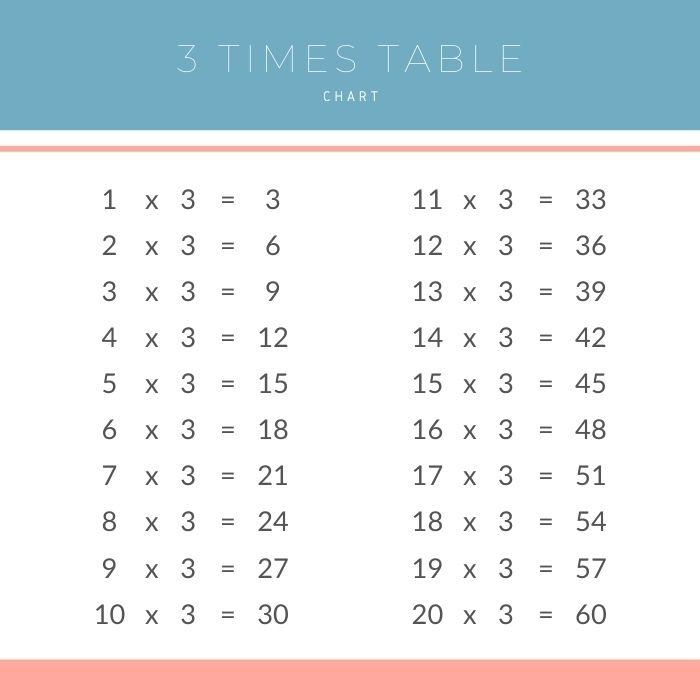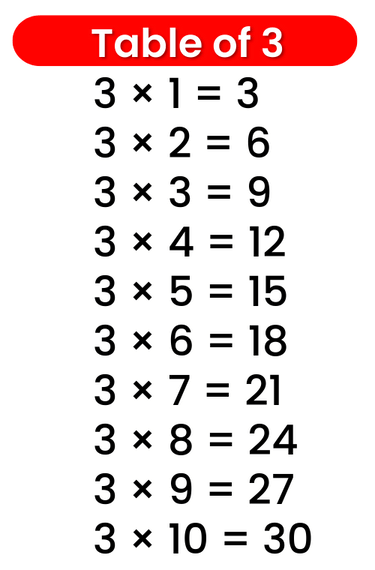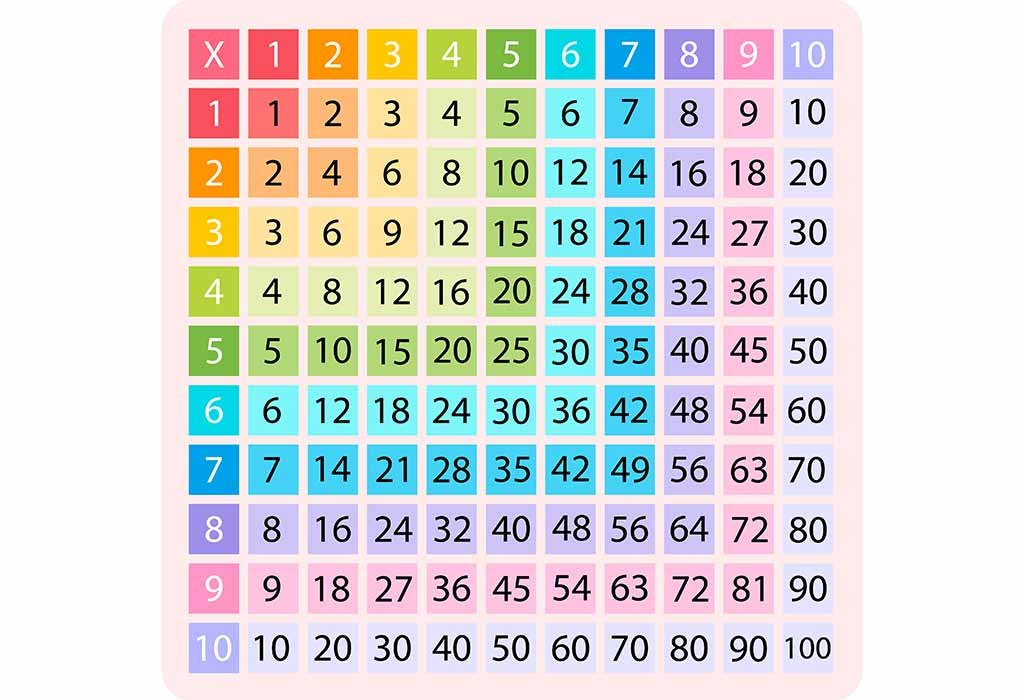# Times Table of 3, 3 ka table

## Times Table of 3, 3 ka table

Maths Multiplication Table of 3, Times Table of 3, 3 ka table, 3 ki Table Chart

3 × ‌1 = 3
3 × ‌2 = 6
3 × ‌3 = 9
3 × ‌4 = 12
3 × ‌5 = 15
3 × ‌6 = 18
3 × ‌7 = 21
3 × ‌8 = 24
3 × ‌9 = 27
3 × ‌10 = 30

## 3 ka table### Multiplication Tables of three upto 20### Times Table of 3 chart upto 10